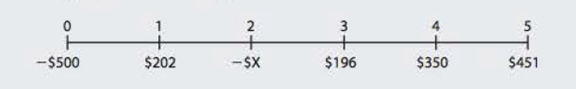Chapter 11, Problem 22P### Fundamentals of Financial Manageme...

9th Edition
Eugene F. Brigham + 1 other
ISBN: 9781305635937

#### Solutions

Chapter
Section### Fundamentals of Financial Manageme...

9th Edition
Eugene F. Brigham + 1 other
ISBN: 9781305635937
Textbook Problem
117 views

# MIRR A project has the following cash flows:This project requires two outflows at Years 0 and 2, but the remaining cash flows are positive. Its WACC is 10%, and its MIRR is 14.14%. What is the Year 2 cash outflow?

Summary Introduction

To compute: The Year 2 cash outflow.

Introduction:

Modified Internal Rate of Return (MIRR): It refers to the rate of return that is computed by the company to make a decision of selection and ranking of a project for investment. This is a modified version of IRR with reinvestment of cash flows at the cost of capital.

Explanation

Given information:

Initial investment of project is $500. Cash inflow at the end of year 1 is$202.

Cash inflow at the end of year 3 is $196. Cash inflow at the end of year 4 is$350.

Cash flow at the end of year 5 is $451. WACC of the project is 10%. MIRR of the project is 14.14%. Terminal value is$1,369 (working note).

The formula to calculate MIRR is,

MIRR=(TerminalValuePresentValue Costs)1N1

Where,

• N is the number of years.

Substitute $1,369 for terminal value, 14.14% for MIRR, ($500+$X) for present value costs and 5 for N in the above formula. 0.1414=($1,369($500+$X))151(1.1414)5=($1,369$500+$X)1.9373=$1,369$500+$X$500+$X=\$706

### Still sussing out bartleby?

Check out a sample textbook solution.

See a sample solution

#### The Solution to Your Study Problems

Bartleby provides explanations to thousands of textbook problems written by our experts, many with advanced degrees!

Get Started